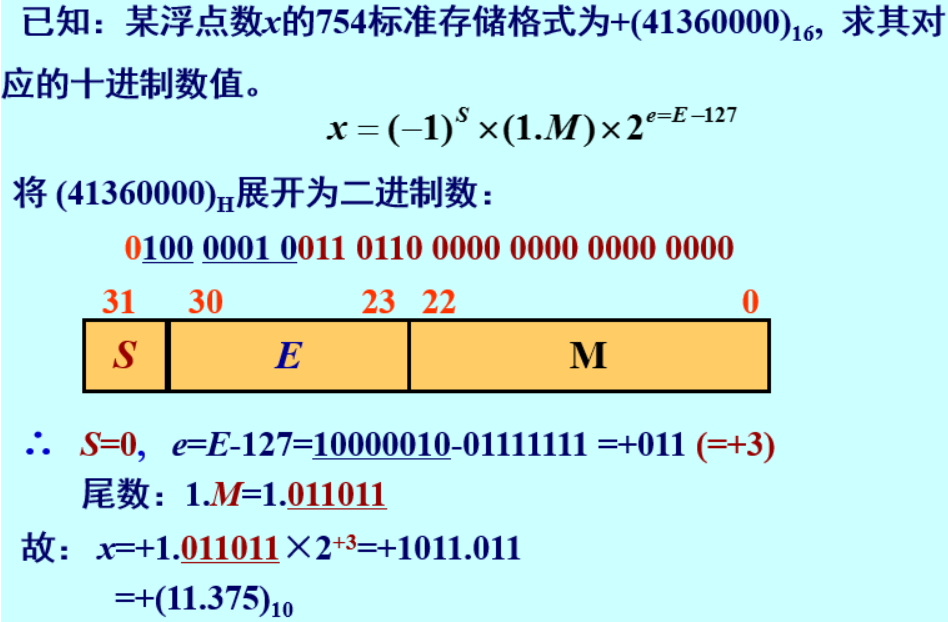# 计算机组成原理复习---第二章 运算方法和运算器

### 文章目录

#### 2.1数制

数的编码表示：原码、反码、补码、移码

数的小数点表示：定点表示、浮点表示。（重点）

其他：BCD码、奇偶校验码、ASCII码等

进位计数数制 基数：数制中所用到的代码的个数；如十进制为0-9基数10，二进制位0、1基数2

权：不同位数的固定常数。

计算机使用二进制原因

1.二进制表示的状态便于物理器件实现；

2.运算规则简单；

3.便于实现逻辑运算 （最好是e进制 约为2.71828）

数制转换举例

D为十进制，O为八进制，H为十六进制，B为二进制 十进制转二进制：(按权相加法)

例：130D = 128 + 2 -->128 = 2^7 and 2=2^1 即 10000010

​ 0.625D = 0.5 + 0.125 -->0.5 = 1/2 = 2^-1 and 0.125 = 1/8 = 2^-3 即0.101

1. 二进制转十进制：(按权相加法)

​ 1001.01B = 1000 +1 +0.01 --> 1000 = 2^3=8 + 1=2^0=1 +0.01=2^-2=1/4=0.25 = 8+1+0.25=9.25D

1. 八进制转二进制：将八进制的每一位都转化为三位对应的二进制，不足补零

2. 十六进制转二进制：将十六进制的每一位转化为四位对应的二进制，不足补零

3. 十六进制转八进制：将十六进制先转化为二进制然后从小数点开始三位一划，转为八进制即可

4. 整数十进制转二进制：除2取余，倒序排列

5. 小数十进制转二进制：乘2取整，顺序排列
例：0.875D 化为二进制

0.875*2=1.75 取1 余0.75

0.75*2=1.5 取1 余0.5

0.5*2 = 1

顺序排列：111 即0.875D = 0.111B

6. 小数二进制转十进制：按位乘以权重即 2^-1 =1/2=0.5,2^-2 =1/4=0.25,2^ -3 =1/8= 0.125…

#### 2.2数值数据的编码

机器数

机器数：数据在机器中的表示

小数点的位置需要约定：定点数和浮点数两类（小数点位置是固定的还是浮动的）

定点机器数的原、反、补码： 正数的原码、反码、补码都是相同的； 负数反码是原码取反，补码：原码取反+1

原码

如 X = +1011 [X]原=0 1011 ，X = - 1011 [X]原= 1 1011

-0.1011 字长为8位 原码：1.1011000

-1011，字长为8位 原码：10001011 即[-1011]原 = 10001011

原码表示，符号位数值化，数值位不变 ，0的原码表示不唯一

原码表示的优点：是直观易懂，机器数和真值之间的相互转换容易，实现乘除的规则简单，

缺点：实现加减运算的规则复杂。

补码

引入目的：将减法变为加法；使符号位能同数值位一起参加运算

正数的补码就是原码，负数的补码取反加一

小数补码得模是2，整数补码的模为2^n+1

例：[X]补 = 110011011 求X？ X = （-1)*2^8+ 1 *2^7+1 *2^4 + 1 *2^3 + 1 *2^1+1 = -256+155 = -101

例：X = -0.1011B [X]原=1.1011B [X]补 =1.1011B

由小数补码的定义，-0.1011B 因为-1< X <0 -->2+X (X为负数，要将符号带着加即为减) 即 10 - 0.1011 -->[X]补 =1.1011B

零的补码表示是唯一的，原码表示是不唯一的

移码

• 移码中0为负，1为正
• 0的移码表示唯一
• 移码与补码仅仅符号位相反其余各位相同
• 移码表示实际是把真值映射到了正数域，可按无符号数比较大小。

移码中逗号不是小数点，是表示左边一位是符号位。

例：当正数 e= +10101 [e]移= 1,10101；当负数 e = -10101 [e]移= 2^5 + e = 2^5 -10101 = 0,01011

#### 2.3数的定点浮点表示

定点数

定点表示：小数点位置隐含的固定

浮点数远比定点数表示的范围大，在单片机中多使用定点表示。

X = Xn Xn-1 Xn-2 …X0

定点数据常用的两种形式：纯小数和纯整数

纯小数的小数点位置约定在Xn的后面，纯整数约定在X0的前面

定点小数 -1 ~ 1

符号位 0为正，1为负，目前计算机中多采用纯整数。

浮点数

浮点数 = 阶码 + 尾数（数据 小数点位置浮动、不固定）+符号

浮点数分为32位浮点数和64位浮点数。

32位浮点数:S为符号位,占一位;E为阶码占用8位,剩下23位为尾数位M.

64位浮点数:S为符号位占1位,E为阶码占11位,剩下52位尾数位M.

符号位：S=0为正数，S=1为负数

若不对浮点数作约束，则同一数据的编码表示不唯一。

如1999 = 1.999 * 10 ^3 =199.9 *10

1011.1101B = 0.10111101*2^100 = 0.0010111101 * 2^110（这里的100和110为二进制，不是十进制）

浮点数规格化：0.5 < 尾数的模 < 1;非规格化数据通过左移尾数和修改阶码来进行规格化。

32位浮点数的规格化表示： x = （-1）^S * (1.M) * 2^(E-127)

64位浮点数的规格化表示： x = （-1）^S * (1.M) * 2^(E-1023)BDC码（有权）

用四位二进制数表示一个十进制数

常见的8421码 2421码 5211码 余3码 格雷码等

总结：

数值型数据（重点）：

机器数、定点数、浮点数、BCD码…

字符型数据：

ASCII码 字符串 汉字等

检错纠错码：

奇偶校验、海明校验、循环冗余校验

逻辑型数据：

0、1

#### 2.4 运算方法

移位运算

移位运算分为逻辑移位 和 算术移位

逻辑移位：整组数据进行移位，本身并不发生变化，只是位置的改变

算术移位：符号位保持不变，数值发生改变

正数的左移右移空位均补0

负数：原码移位后都补0，反码移位后补1，补码左移补0，右移补1

定点加减法运算

计算机中不使用原码做加减，原码一般用来做乘除运算，一般使用补码来做加减法运算

补码的加法公式 [X]补 +[Y]补 = [X+Y]补

1. 相加两数都是正数，结果一定为正数
2. 相加两数一正一父，结果不确定
3. 相加两数为负数，结果一定为负数

例：x = +1001 y=+0101 求x+y

X补 = 01001 ，Y补=00101 [X+Y]补=01110 =+1110

溢出的位丢掉

补码减法公式：[X-Y]补= [][][X]补 - [Y]补 = [X]补 + [-Y]补

例：X=+1101 Y = +0110 求 X-Y

X补 = 01101 Y补=00110 -Y补 = 11010

X-Y 补=100111 = 00111 = +0111

溢出

正溢：两个正数相加，结果大于机器字长所能表示的最大正数

负溢：两个负数相加，结果小于机器所能表示的最小负数

两种检测方式：

• 双符号位法

用00替换掉原来的0表示正数，11替换掉1表示负数，这样机器码所能表示的数范围扩大一倍。

如果进行加法运算后，符号位仍是00或11则代表没有溢出，当出现01、10时代表出现溢出，此时取最高位作为符号位。

• 单符号位法

#### 2.5 定点运算器的组成

运算器是数据加工处理的部件，是CPU的重要组成部分。

最基本的结构：算术/逻辑运算单元，数据缓冲寄存器，通用寄存器，多路转换器，数据总线等

逻辑运算：逻辑非，逻辑加，逻辑乘，逻辑异

逻辑非：取数的相反数，类似于负数的反码，逻辑非用变量上方加一条横线表示

例：X=101011 [X]非 = 010100

逻辑加：也叫逻辑或，按位求或 用 + 表示 ，有1为1

例：X=100101 Y= 110011

​ 100101

​ 110011

= 110111

逻辑乘：按位进行与运算，也叫逻辑与 用 · 表示 ，有0为0

例：X=1010011 Y=1100101

​ 1010011

​ 1100101

= 1000001

逻辑异：按位加，相同为0不同为1
例： x=10101011 y=11001100

​ 10101011

​ 11001100

= 01100111

多功能算术/逻辑运算单元ALU

总线：计算机内部的主要工作是信息传送和加工的过程，因此机器内部各部件之间的数据传送非常频繁。为了减少内部数据线，通常将寄存器之间数据线加以归并，形成了总线结构。

内部总线：CPU内各部件之前连线

外部总线：系统总线，CPU和存储器、IO设备之间的连线。

定点运算器的基本结构

• 单总线结构的运算器：所有的部件都接到同一总线上，数据可以在任意寄存器和ALU之间传送；同一时间，只允许一个操作数放在单总线上。速度慢，结构简单。
• 双总线结构的运算器：用两条总线来连接部件，专用寄存器一分为二分别连接两个总线，此时两个操作数的运算只需要一次操作控制。
• 三总线结构的运算器：在双总线的基础上，新增一个总线用来连接通用寄存器和ALU，ALU输出连接到第三条总线。

#### 2.6 浮点数做加减

需要其阶码相等，也采用补码得形式进行加减法运算

• 0操作数检查 如果两个操作数x和y中有一个数为0就不需要进行下面的操作。

• 对阶–小阶向大阶看齐（通过移动尾数来进行，右移阶码增加尾数精度损失，左移阶码减少尾数高位损失）

X = 0.111010 *2^011 [X]补=00，011；00.111010

Y = -0.111011 *2^110 [Y]补=00，110;11.000101

X向Y看齐

[X]补=00，110；00.000111

[Y]补=00，110；11.000101 -[Y]补=00,110;00.111011

• 阶码相同，只对尾数部分求和

X+Y = 00,110;11.001100（已规格化）

X-Y = 00,110;01.000010（需要规格化处理）–》00，110；00.10001（已规格化）

• 进行尾数的移动实现规格化

01.XXX 一般采用右规–》00.1XX

10.XXX --》11.0XX

• 舍入 0舍1入：{丢掉数位为0则舍去，丢掉数位为1，则尾数末尾加1}； 末位恒置1：只要有数位被移掉，末尾就置为1
• 溢出处理：阶码符号位相异就会溢出
例： x=0.5D y= -0.4375D  求 x+y 浮点数
#首先将转换为二进制数
x= 0.5D = 0.1B = 1.000B*2^-1  y= -0.4375D = -0.0111B = -1.110B*2^-2
#对阶  y的阶小，小阶向大阶看齐,2^-1
x= 1.000B*2^-1  y= -0.111B*2^-1
#尾数求和
x+y =  1.000B*2^-1 + (-0.111B*2^-1 ) = 0.001B*2^-1
#规格化处理
x+y = 0.001B*2^-1 = 1.000B*2^-4
#检查溢出    127 >= -4 <= -126  没有溢出
#舍入操作
1.000B*2^-4  尾数有效位刚好4位，舍入不需改变。
1.000B*2^-4 = 0.0625D


浮点运算流水线

计算机的流水线处理过程和工厂中流水线类似。

为了实现流水，需要将任务分割成一系列的子任务，使得各个子任务可以在流水线的各个阶段可以并发地执行。将任务连续不断的输入流水线，从而实现子任务级的并行。实现了时间并行性。

在流水线中，原则上要求各个阶段的处理时间都相同。

例：假设有一个4级流水浮点加法器每个过程段所需要的时间为：0操作数检查t1 = 70ns，对阶t2=60ns，相加t3=90ns，规格化t4=80ns，缓冲寄存器L的延时为t5=10ns。

​		 求：1.加法器的流水线时钟周期至少为：

​				t = 90ns + 10ns = 100ns

​			 不是流水方式：时间t=t1 + t2+ t3+ t4 = 300ns
4级流水线的加速比： Ck = 300/100 = 3

​			  2.每个过程段的时间都是75ns，求加速比

​			Ck = 300/75 = 4



08-14778

#### 【连载】计算机组成原理 --- 第二章数据的表示和运算还能输入1000个字符

11-269416

#### 计算机组成原理：运算方法和运算器

06-2133

#### 计算机组成原理--期末复习--第二章

04-19196

#### 计算机体系结构——流水线技术（Pipelining）

07-01108

#### 计算机组成原理(科学出版社) 第二章：运算方法和运算器

03-256937

#### 浮点流水线运算

06-14114

#### 计算机组成原理习题 第二章 运算方法和运算器

04-023010

#### Modelsim 第一个仿真程序 四位加法器

07-061851

#### 【计算机组成与设计/硬件软件接口】第四章 处理器(流水线，中断，指令级并行）

05-17789

#### 计算机组成原理复习题二

06-20211

#### 计算机组成原理 — 后四章（期末复习版）

06-1212万+

#### 全面理解Java内存模型(JMM)及volatile关键字

08-27861

#### 优化基于FPGA的深度卷积神经网络的加速器设计

07-172938

#### Verilog HDL小练习（一）二路选择器&&三位加法器

03-31697

#### 计算机组成原理学习笔记——指令流水线

02-264526

#### ﻿﻿﻿﻿基于DLX指令集的5级流水线CPU设计与实现©️2020 CSDN 皮肤主题: 黑客帝国 设计师: 上身试试
实付0元点击重新获取扫码支付
钱包余额 0抵扣说明：

1.余额是钱包充值的虚拟货币，按照1:1的比例进行支付金额的抵扣。
2.余额无法直接购买下载，可以购买VIP、C币套餐、付费专栏及课程。余额充值